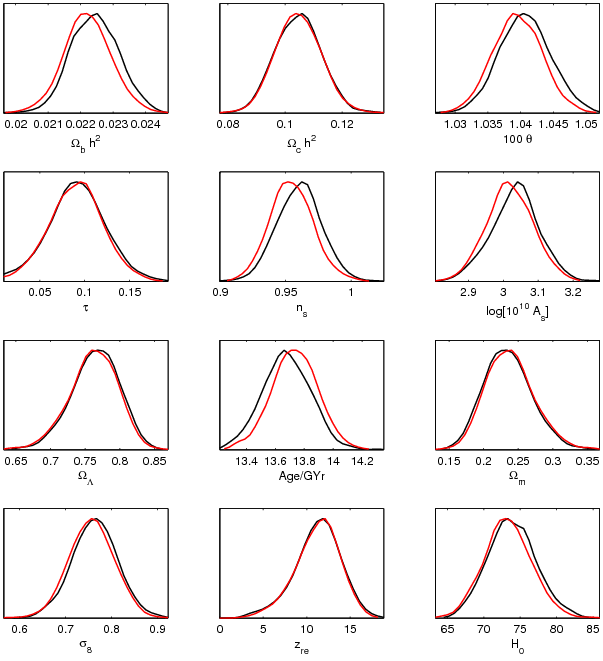## WMAP likelihood approximations

Use of Healpix, camb, CLASS, cosmomc, compilers, etc.
Antony Lewis
Posts: 1609
Joined: September 23 2004
Affiliation: University of Sussex
Contact:

### WMAP likelihood approximations

In astro-ph/0603587 (page 17) the authors discuss some options in the WMAP likelihood code. In particular they say that the default code uses a Gaussian approximation for the C_l^T likelihood. I presume this is referring to the beam calculation only - WMAP_3yr_likelihood.f90 appears to be using a sum of lognormal and normal as in the first-year code for the main calculation on non-large scales.

The beam option variables seem to be defined in WMAP_3yr_tt_beam_and_ptsrc_corr.f90, in contradiction to the README.txt statement
The options/parameters for use in the code are all contained the WMAP_options module.

Could someone please clarify what these various beam options are, and what effect they have on the results?

Anze Slosar
Posts: 183
Joined: September 24 2004
Affiliation: Brookhaven National Laboratory
Contact:

### WMAP likelihood approximations

Just a snippet from Hiranya's e-mail explaining this and which was forwarded to me (I am sure Hiranya wouldn't mind):

The difference of my analysis from the shipped code is just in the
treatment of how beam errors are propagated in the likelihood function, as described in Appendix A.2 of Hinshaw et al (2006). I use the full off
diagonal TT covmat, Gauss+ln normal likelihood, and fixed fiducial Cls to
propagate the beam errors. The official release has the beam module using
diagonal TT covmat, Gaussian likelihood, and varied theory cls. You can
recover the beam error treatment I am using by setting
beam_fixed_fiducial_spectrum = .true.
beam_diagonal_sigma = .false.
beam_gaussian_likelihood=.false.
in WMAP_3yr_tt_beam_and_ptsrc_corr.f90

I would like to emphasize that there is no error in the shipped code. I
needed the greater accuracy of the gauss+ln normal treatment in order to
deal with some models I am trying to constrain which allow much greater
freedom in the primordial power spectrum.

Antony Lewis
Posts: 1609
Joined: September 23 2004
Affiliation: University of Sussex
Contact:

### Re: WMAP likelihood approximations

Thanks.

Here's a comparison plot comparing WMAP3 six parameter with the default (red) compared to with those options (black).Anze Slosar
Posts: 183
Joined: September 24 2004
Affiliation: Brookhaven National Laboratory
Contact:

### Re: WMAP likelihood approximations

Antony Lewis wrote:Thanks.

Here's a comparison plot comparing WMAP3 six parameter with the default (red) compared to with those options (black).
Good, so it is indeed completelly negligible, except maybe for a very marginal increase in n_s=1 tail of n_s...

Hiranya Peiris
Posts: 54
Joined: September 28 2004
Affiliation: University College London

### WMAP likelihood approximations

I just noticed this posting. The change in beam treatment only causes slight changes as Antony has seen, and I have updated astro-ph/0603587 to clarify this point.

Cheers
Hiranya

Loison Hoi
Posts: 23
Joined: February 21 2006
Affiliation: McGill University

### WMAP likelihood approximations

Hi,

Maybe the followings are related to this topic.

I accidentally found a best fit point of the running spectral inex model (6 parameters + tensor + running spectral index) which gives \chi^2 = 10604 when using cosmomc. Compared with the best fit non-running model (6 parameters) value \chi^2=11252, there is a \Delta\chi^2 = 648! This is a huge change in likelihood. The values of parameters of this special best fit point are very different from those of the usual best fit point, for example dn/dlnk is positive, and hence this special point does not seem physical. Here are the values of parameters of the special best fit point when using cosmomc (inflation_consistency=T, other settings are default):

Best fit sample -log(Like) = 5302.02880859375
param bestfit
1 0.168166E-01 \Omega_b h^2
2 0.133521E+00 \Omega_c h^2
3 0.104458E+01 \theta
4 0.644379E-01 \tau
8 0.593603E+00 n_s
10 0.676360E-01 n_{run}
11 0.270513E+01 log[10^{10} A_s]
12 0.948667E+00 r

With detailed investigation, I found that the main question is that the TT beam and point source correction is very close to the TT C_l's likelihood,
but with the opposite sign, and thus the total likelihood is reduced significantly. Here are the likelihoods:

likelihoods for the special best fit point:
-2*ln(L)_ttlike = 114373.747999890
-2*ln(L)_ttbeamlike = -114373.598731511
-2*ln(L)_ttlowlike = 602.164159554811
-2*ln(L)_ttlowdet = -10999.7907555282
-2*ln(L)_telike = 1197.98368076825
-2*ln(L)_tedet = 3401.19832251655
-2*ln(L)_lowlike = 1190.72692871094
-2*ln(L)_lowdet = 15211.7269109651
Likelihood: 5302.079

likelihoods for the usual running spectral index model:
-2*ln(L)_ttlike = 1052.37469675378
-2*ln(L)_ttbeamlike = 1.46484474064230
-2*ln(L)_ttlowlike = 640.235204763192
-2*ln(L)_ttlowdet = -11040.4322809536
-2*ln(L)_telike = 417.149844866212
-2*ln(L)_tedet = 3776.02275889830
-2*ln(L)_lowlike = 1180.03723144531
-2*ln(L)_lowdet = 15222.2984174593
Likelihood: 5624.575

Therefore, we can see that every thing looks normal except for the beam and point source corrections. Michael Nolta, the author of the code WMAP_3yr_tt_beam_and_ptsrc_corr.f90, informed me that to fix this problem, we can try using the following options:

beam_diagonal_sigma = .false.
beam_gaussian_likelihood = .false.

This will integrate the beam module into the gaussian+lognormal form for the likelihood used for the TT spectrum, instead of using a gaussian approximation. Anyway, maybe the gaussian approximation for the likelihood is not valid in some regions, for example dn/dlnk>0 as in the above example.

By the way, there is a bug in the function "compute_tt_beam_and_ptsrc_chisq" in WMAP_3yr_tt_beam_and_ptsrc_corr.f90. This function defines the dimensions of C_l's arrays and the fisher matrix through the input lmin and lmax, which makes the C_l's and the fisher matrix inside the function different from those of the input values if lmin/=2 and lmax/=1000. For example, if lmin=3 and lmax=1000, according to the Fortran data passing rule, we have

cl(2) = cl(3)
cl(3) = cl(4)
cl(4) = cl(5)
fisher(2,2) = fisher(3,3)
fisher(3,3) = fisher(5,4)
fisher(4,3) = fisher(6,4)

where values in the left hand side are values outside the function (e.g. in the subroutine pass2_compute_likelihood), and values in the right hand
side are values inside the function compute_tt_beam_and_ptsrc_chisq. Problems will occur in the beam and point source corrections in TT data if
ttmin and ttmax are not set to the default values, 2 and 1000. To avoid this problem, just set the dimensions of the arrays and matrix:

real(kind=8), dimension(2:1000), intent(in) :: cltt, cltt_dat, neff, fsky, clps, z, zbar
real(kind=8), dimension(2:1000,2:1000), intent(in) :: fisher

Loison Hoi
22 Jun 2006